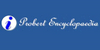### tractrix<geometry> A curve such that the part of the tangent between the point of tangency and a given straight line is constant; so called because it was conceived as described by the motion of one end of a tangent line as the other end was drawn along the given line. ... (11 Mar 1998) ...
Found on http://www.encyclo.co.uk/local/20973

### Tractrix• (n.) A curve such that the part of the tangent between the point of tangency and a given straight line is constant; -- so called because it was conceived as described by the motion of one end of a tangent line as the other end was drawn along the given line.
Found on http://thinkexist.com/dictionary/meaning/tractrix/

### tractrixA curve, sometimes called the trajectory curve or equitangential curve, that is the answer to a question asked by the Frenchman Claude Perrault (1613-1688). Perrault is not a giant in the annals of mathematics; in fact, he trained as a doctor and gained a minor reputation as an architect and an anat...
Found on http://www.daviddarling.info/encyclopedia/T/tractrix.html

### TractrixA tractrix is a curve whose tangents are all of equal lengths, the involute of a catenary.
Found on http://www.probertencyclopaedia.com/browse/GT.HTM

### TractrixTractrix (from the Latin verb trahere `pull, drag`; plural: tractrices) is the curve along which an object moves, under the influence of friction, when pulled on a horizontal plane by a line segment attached to a tractor (pulling) point that moves at a right angle to the initial line between the object and the puller at an infinitesimal speed. I.....
Found on http://en.wikipedia.org/wiki/Tractrix
No exact match found